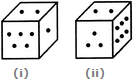# Non Verbal Reasoning - Cubes and Dice - Discussion

### Discussion :: Cubes and Dice - Section 2 (Q.No.13)

13.

Two positions of a block are given below. When 1 is at the top, which number will be at the bottom?[A]. 2 [B]. 3 [C]. 4 [D]. 6

Explanation:

Number 2 is common to the two positions of the dice. We assume the dice in fig. (ii) to be rotated so that 2 remains on the top face (i.e. face V as per activity 1) and the numbers 3 and 6 move to the faces hidden behind 5 and 1 respectively [in fig. (i)]. Then, clearly, 5 lies opposite 3 and 6 lies opposite 1. Hence, when 1 is at the top, then 6 will be at the bottom.

 Raw said: (Oct 15, 2015) Sum of dots on opposite faces is 7 so the face opposite 1 dot has 6 dots. So when 1 is on top 6 is at the bottom. Figure (ii) is not even required to answer this question.

 Liz said: (May 23, 2021) @Raw. Then why it doesn't apply to the number 37? Explain please.